### Home > CCA > Chapter Ch8 > Lesson 8.1.3 > Problem8-30

8-30.
1. Remember that a square is a rectangle with four equal sides. Homework Help ✎

1. If a square has an area of 81 square units, how long is each side?

2. Find the length of the side of a square with area 225 square units.

3. Find the length of the side of a square with area 10 square units.

4. Find the area of a square with side 11 units.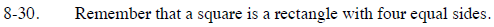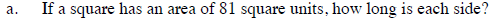Take the square root of the area to find the length of each side.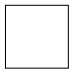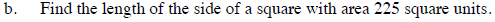Use the same strategy as part (a).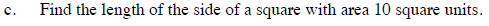Use the same strategy as part (a).

$\text{\sqrt{10}\,units}$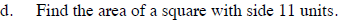Area = (length)(width)